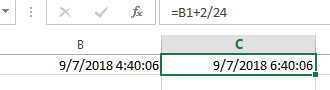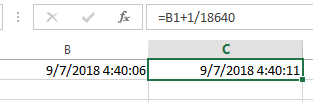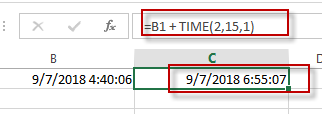# Adding Hours, Minutes, or Seconds to a Date and Time in Excel

This post will guide you how to add hours, minutes, or seconds to a given date and time in a cell in Excel. How do I add hours, minutes or seconds to a time with a formula in Excel. How to add hours to a date and time in Excel. How do I add 15 minutes to a time in excel.

## Add Hours to a Date and Time

If you want to add 2 hours to a specific date and time in one cell, you can use the following formula to achieve the result.

`=B1+ 2/24 `

You need to type this formula into a blank cell and then press Enter key in your keyboard, and drag the AutoFill Handle over other cells to apply this formula to add 2 hours for other dates.This formula will return a serial number, so you need to format the serial number as the standard date and time format.

## Add Minutes to a Date and Time

If you want to add 15 minutes to a date and time in one cell, you can use the following formula:

`=B1 + 15/1440`

## Add Seconds to a Date and Time

If you want to add 1 second to a date and time in one cell, you can use the following formula:

`=B1 + 1/18640`You can also create another formula based on the TIME function to achieve the same result.
For example, you want to add 2 hours, 15 minutes, 1 second to a data and time value in Cell B1, you can use the following formula:

`=B1 + TIME(2,15,1)`